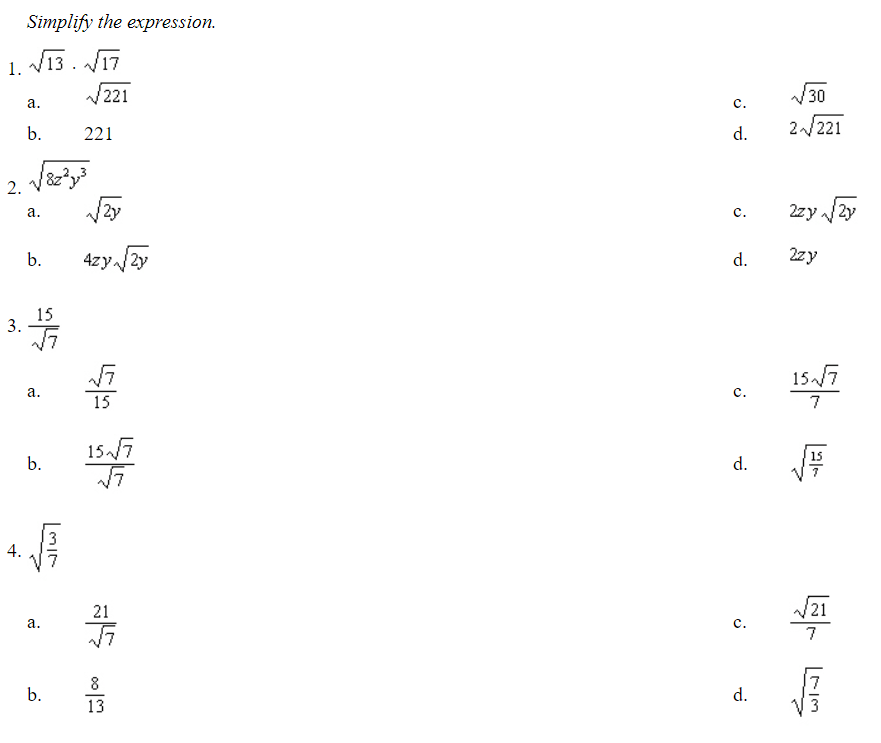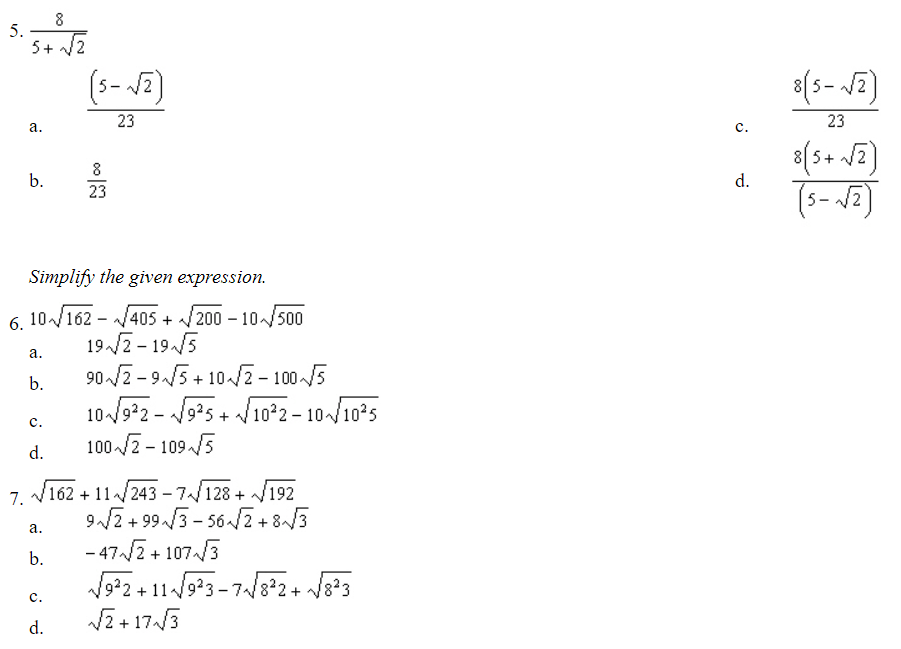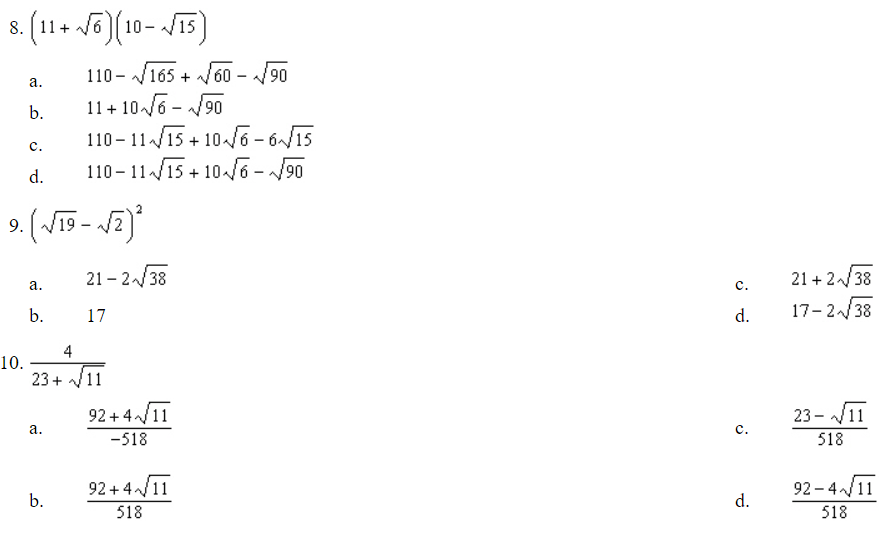# Radical ExpressionsAnswers (A, C, C, C, C, D, B, D, A, D)

Answer explanations are below.

1. Use the product property of square roots. Then simplify the result.
2. Find the prime factorization of the radicand. Use the Product Property and simplify the result.
3. Multiply by a form of one to remove the radical expression from the denominator. Simplify the result.
4. The square root of the quotient is equal to the quotient of each square root. Multiply by a form of one to remove the radical expression from the denominator. Simplify the result.
5. Find the conjugate of the denominator. Multiply by a form of one that includes the conjugate. Simplify the result.
6. Factor each term using squares and use the Product Property of Radicals. Then, combine the similar radicals to obtain a simplified expression.
7. Factor each term using squares and use the Product Property of Radicals. Then, combine the similar radicals to obtain a simplified expression.
8. Use the FOIL method to multiply the radicals and use the Product Property of Radicals to simplify the expression.
9. Use the FOIL method to multiply the radicals and use the Product Property of Radicals to simplify the expression.
10. Multiply the numerator as well as the denominator by the conjugate of the denominator. Use the FOIL method and the difference of squares to simplify the given expression.

(source)